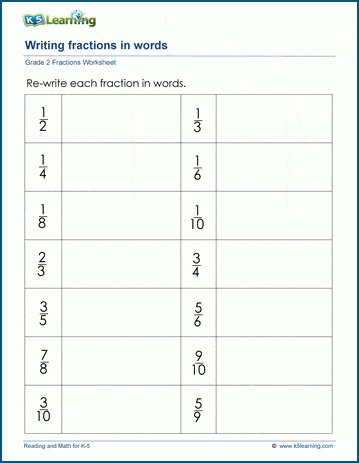# Writing fractions in words

## Fraction Worksheets: fractions in words

These worksheets reinforce the equivalence between fractions represented with a numerator and denominator (e.g. "1/2") and the same fraction in words ("one half"); students need to gain fluency in both form of expressing fractions.## More fractions worksheets

Explore all of our fractions worksheets, from dividing shapes into "equal parts" to multiplying and dividing improper fractions and mixed numbers.

## What is K5?

K5 Learning offers reading and math worksheets, workbooks and an online reading and math program for kids in kindergarten to grade 5.  We help your children build good study habits and excel in school.